# Scaled Transfer Function Estimator

May 24, 2019

The scaled transfer function estimator Ĥs(f) is an estimate of the true transfer function of a system with scaling factor s, where 0 ≤ s ≤ ∞.

The scaling factor s is the ratio of output noise power to input noise power such. Ŝ = Ŝyy / Ŝxx, assuming Ŝxx(f) and Ŝyy(f) are constant with respect to frequency f (x and y are white noise sequences).

Ĥs is a generalization of the transfer function estimators Ĥ1, Ĥ2, and Ĥv.

Ĥs is defined as:

(1)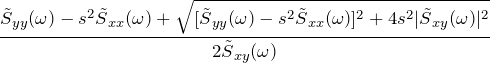### Alternate Forms of Ĥs

Using the technique of “rationalizing the denominator” (or in this case, “rationalizing the numerator”), we can arrive at alternate useful forms for Ĥs. In particular, if we multiply numerator and denominator by the rationalizing factor,

(2)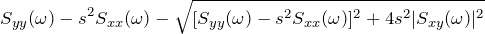we can arrive at the equation:

(3)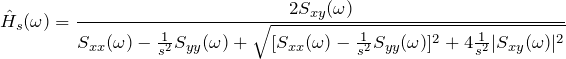Moreover, if we divide the numerator and denominator by s², we get:

(4)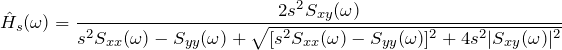### Special Cases of Ĥs

Ĥ1 , Ĥ2 and Ĥ are special cases of Ĥs. Using the definition of Ĥs, we see

• Ĥs=0 = Ĥ2
• Ĥs=1 = Ĥy

Using the second alternate form, we see Ĥs→∞ = Ĥ1.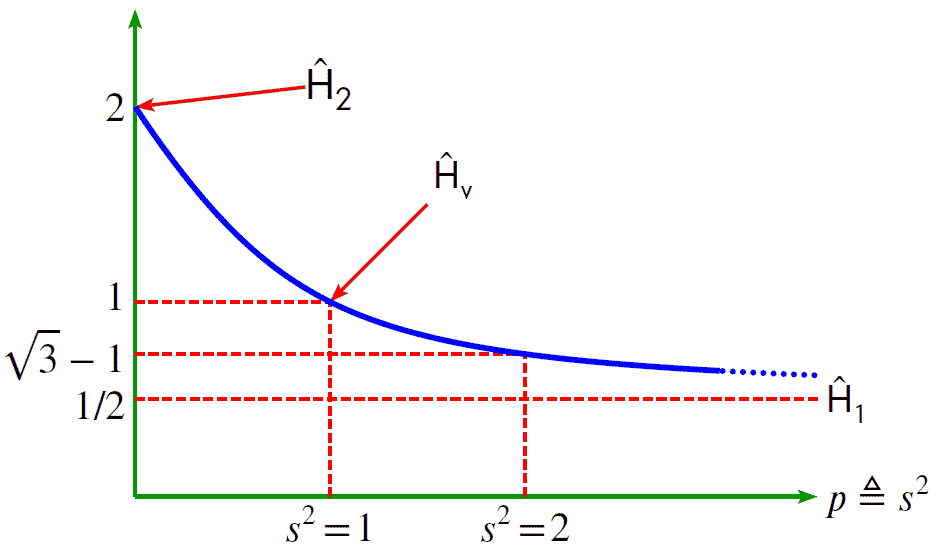Figure 1.5. Transfer function, H, vs. the scaling factor, s, squared.

### MATLAB® Resources

 pwelch(…) Estimate power spectral density. https://www.mathworks.com/help/signal/ref/pwelch.html cpsd(…) Estimate cross power spectral density. https://www.mathworks.com/help/signal/ref/cpsd.html

#### Example of MATLAB Code

clear                    % clear all variables
format long g;           % set format of text output
N       = 16;            % length of input data sequence
Fs      = 1;             % sample rate (used for scaling)
percent = 50;            % percent overlap
Nfft = N * (1-percent/100);
x= [0 0 0 0 0 0 0 0 0 0 0 1 1 1 0 0]’;
y= [0 0 0 1 1 1 1 1 0 0 0 0 0 0 0 0]’;
%—————————————————————————
% smoothing window
%—————————————————————————-
window = hanning(Nfft);   % Hanning     window
%—————————————————————————-
% calculate estimates
%—————————————————————————-
[Syx,freq]=cpsd(      x,y,window,Noverlap,Nfft,Fs); % estimate Syx
[Sxx,freq]=pwelch(    x,  window,Noverlap,Nfft,Fs); % estimate Sxx
[Syy,freq]=pwelch(      y,window,Noverlap,Nfft,Fs); % estimate Syy>
% |     |         |              |   |  |              |                |       |______ sample rate
% |     |         |             |    |  |              |                |__________ estimate length
% |     |         |             |    |  |              |___________________ num. overlapping positions
% |     |         |             |    |  |___________________________ window type
% |     |         |             |    |_____________________________ data sequence y
% |     |         |             |_______________________________ data sequence x
% |     |         |_______________________________________ estimation operation
% |     |_____________________________________________ x-axis sequence
% |________________________________________________ estimate sequence
%—————————————————————————-
% estimate scaling parameters
%—————————————————————————-
s = var(y)/var(x) % this is ‘not’ a recommended way to estimate s!
%—————————————————————————-
% estimate Hs
%—————————————————————————-
Hs = estimate_Hs(s,Sxx,Syy,Sxy);
%—————————————————————————-
% function to estimate Hs
%—————————————————————————-
function Hs = estimate_Hs(s,Sxx,Syy,Sxy)
u1 = Syy;
u2 = s * Sxx;
u3 = (s * Sxx – Syy) .* (s * Sxx – Syy);
u4 = abs(Sxy) .* abs(Sxy);
v1 = 2.0 * Sxy;
Hs = (u1 – u2 + sqrt(u3 + 4 * s * u4)) ./ v1;
end